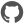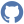# Shape

Namespace: XPlot.Plotly
Parent Module: Graph

### Constructors

 Constructor Description ``` new() ``` Signature: unit -> ShapeCompiledName: `.ctor`

### Instance members

 Instance member Description ``` fillcolor() ``` Signature: unit -> stringSets the color filling the shape's interior. CompiledName: `set_fillcolor` ``` fillcolor() ``` Signature: unit -> unitSets the color filling the shape's interior. CompiledName: `get_fillcolor` ``` line() ``` Signature: unit -> LineCompiledName: `set_line` ``` line() ``` Signature: unit -> unitCompiledName: `get_line` ``` opacity() ``` Signature: unit -> floatSets the opacity of the shape. CompiledName: `set_opacity` ``` opacity() ``` Signature: unit -> unitSets the opacity of the shape. CompiledName: `get_opacity` ``` path() ``` Signature: unit -> stringFor `type` path - a valid SVG path but with the pixel values replaced by data values. There are a few restrictions / quirks only absolute instructions, not relative. So the allowed segments are: M, L, H, V, Q, C, T, S, and Z arcs (A) are not allowed because radius rx and ry are relative. In the future we could consider supporting relative commands, but we would have to decide on how to handle date and log axes. Note that even as is, Q and C Bezier paths that are smooth on linear axes may not be smooth on log, and vice versa. no chained "polybezier" commands - specify the segment type for each one. On category axes, values are numbers scaled to the serial numbers of categories because using the categories themselves there would be no way to describe fractional positions On data axes: because space and T are both normal components of path strings, we can't use either to separate date from time parts. Therefore we'll use underscore for this purpose: 2015-02-21_13:45:56.789 CompiledName: `set_path` ``` path() ``` Signature: unit -> unitFor `type` path - a valid SVG path but with the pixel values replaced by data values. There are a few restrictions / quirks only absolute instructions, not relative. So the allowed segments are: M, L, H, V, Q, C, T, S, and Z arcs (A) are not allowed because radius rx and ry are relative. In the future we could consider supporting relative commands, but we would have to decide on how to handle date and log axes. Note that even as is, Q and C Bezier paths that are smooth on linear axes may not be smooth on log, and vice versa. no chained "polybezier" commands - specify the segment type for each one. On category axes, values are numbers scaled to the serial numbers of categories because using the categories themselves there would be no way to describe fractional positions On data axes: because space and T are both normal components of path strings, we can't use either to separate date from time parts. Therefore we'll use underscore for this purpose: 2015-02-21_13:45:56.789 CompiledName: `get_path` ``` ShouldSerializefillcolor() ``` Signature: unit -> bool``` ShouldSerializeline() ``` Signature: unit -> bool``` ShouldSerializeopacity() ``` Signature: unit -> bool``` ShouldSerializepath() ``` Signature: unit -> bool``` ShouldSerializetype() ``` Signature: unit -> bool``` ShouldSerializex0() ``` Signature: unit -> bool``` ShouldSerializex1() ``` Signature: unit -> bool``` ShouldSerializexref() ``` Signature: unit -> bool``` ShouldSerializey0() ``` Signature: unit -> bool``` ShouldSerializey1() ``` Signature: unit -> bool``` ShouldSerializeyref() ``` Signature: unit -> bool``` type() ``` Signature: unit -> objSpecifies the shape type to be drawn. If line, a line is drawn from (`x0`,`y0`) to (`x1`,`y1`) If circle, a circle is drawn from ((`x0`+`x1`)/2, (`y0`+`y1`)/2)) with radius (|(`x0`+`x1`)/2 - `x0`|, |(`y0`+`y1`)/2 -`y0`)|) If rect, a rectangle is drawn linking (`x0`,`y0`), (`x1`,`y0`), (`x1`,`y1`), (`x0`,`y1`), (`x0`,`y0`) If path, draw a custom SVG path using `path`. CompiledName: `set_type` ``` type() ``` Signature: unit -> unitSpecifies the shape type to be drawn. If line, a line is drawn from (`x0`,`y0`) to (`x1`,`y1`) If circle, a circle is drawn from ((`x0`+`x1`)/2, (`y0`+`y1`)/2)) with radius (|(`x0`+`x1`)/2 - `x0`|, |(`y0`+`y1`)/2 -`y0`)|) If rect, a rectangle is drawn linking (`x0`,`y0`), (`x1`,`y0`), (`x1`,`y1`), (`x0`,`y1`), (`x0`,`y0`) If path, draw a custom SVG path using `path`. CompiledName: `get_type` ``` x0() ``` Signature: unit -> objSets the shape's starting x position. See `type` for more info. CompiledName: `set_x0` ``` x0() ``` Signature: unit -> unitSets the shape's starting x position. See `type` for more info. CompiledName: `get_x0` ``` x1() ``` Signature: unit -> objSets the shape's end x position. See `type` for more info. CompiledName: `set_x1` ``` x1() ``` Signature: unit -> unitSets the shape's end x position. See `type` for more info. CompiledName: `get_x1` ``` xref() ``` Signature: unit -> objSets the shape's x coordinate axis. If set to an x axis id (e.g. x or x2), the `x` position refers to an x coordinate If set to paper, the `x` position refers to the distance from the left side of the plotting area in normalized coordinates where 0 (1) corresponds to the left (right) side. CompiledName: `set_xref` ``` xref() ``` Signature: unit -> unitSets the shape's x coordinate axis. If set to an x axis id (e.g. x or x2), the `x` position refers to an x coordinate If set to paper, the `x` position refers to the distance from the left side of the plotting area in normalized coordinates where 0 (1) corresponds to the left (right) side. CompiledName: `get_xref` ``` y0() ``` Signature: unit -> objSets the shape's starting y position. See `type` for more info. CompiledName: `set_y0` ``` y0() ``` Signature: unit -> unitSets the shape's starting y position. See `type` for more info. CompiledName: `get_y0` ``` y1() ``` Signature: unit -> objSets the shape's end y position. See `type` for more info. CompiledName: `set_y1` ``` y1() ``` Signature: unit -> unitSets the shape's end y position. See `type` for more info. CompiledName: `get_y1` ``` yref() ``` Signature: unit -> objSets the annotation's y coordinate axis. If set to an y axis id (e.g. y or y2), the `y` position refers to an y coordinate If set to paper, the `y` position refers to the distance from the bottom of the plotting area in normalized coordinates where 0 (1) corresponds to the bottom (top). CompiledName: `set_yref` ``` yref() ``` Signature: unit -> unitSets the annotation's y coordinate axis. If set to an y axis id (e.g. y or y2), the `y` position refers to an y coordinate If set to paper, the `y` position refers to the distance from the bottom of the plotting area in normalized coordinates where 0 (1) corresponds to the bottom (top). CompiledName: `get_yref`